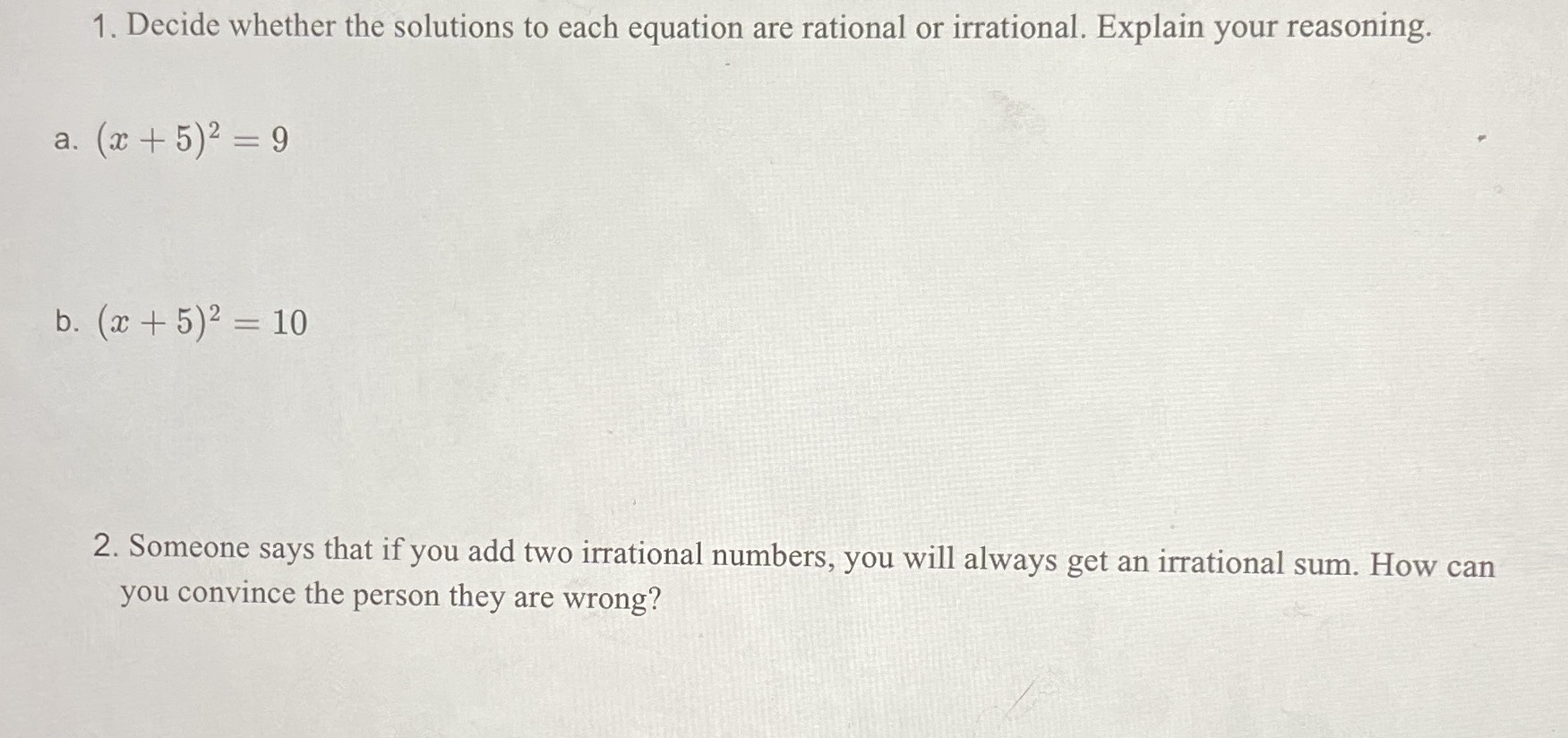### ¿Todavía tienes preguntas de matemáticas?

Pregunte a nuestros tutores expertos
Algebra
Pregunta1. Decide whether the solutions to each equation are rational or irrational. Explain your reasoning.

a. $$( x + 5 ) ^ { 2 } = 9$$

b. $$( x + 5 ) ^ { 2 } = 10$$

2. Someone says that if you add two irrational numbers, you will always get an irrational sum. How can you convince the person they are wrong?